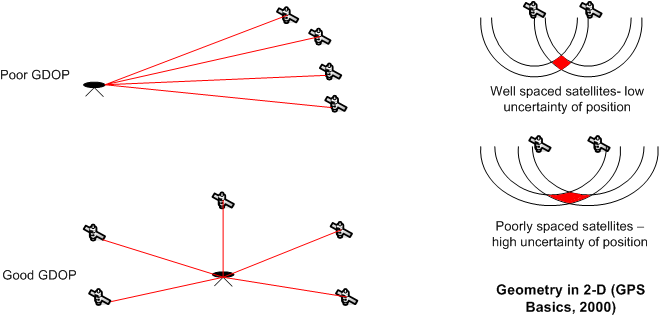# What is GDOP or Geometric Dilution of Precision ?

The idea of Geometric DOP is to state how errors in the measurement will affect the final state estimation.  Geometric Dilution of Precision  Estimate of satellite conditions for a given location & time, Given in distance units (meters or feet), When visible navigation satellites are close together in the sky, the geometry is said to be weak and the DOP value is high; when far apart, the geometry is strong and the DOP value is low. Consider two overlapping ring  of different centres . If they overlap at right angles, the greatest extent of the overlap is much smaller than if they overlap in near parallel. Thus a low DOP value represents a better positional precision due to the wider angular separation between the satellites used to calculate a unit’s position.

###### Note:

Ideal GDOP: One Satellite directly overhead w/an abundance of additional satellites spaced evenly around the sky.### 1 Comment

•VIKALP RAWAT says:

What is the ideal value of GDOP one need to check for a better fix